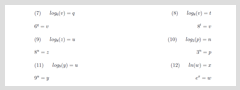# Home | Mathematics | Precalculus

## Precalculus

Exponential functions are used for many real-world applications such as finance, forensics, computer science, and most of the life sciences. Working with an equation that describes a real-world situation gives us a method for making predictions. Most of the time, however, the equation itself is not enough. We learn a lot about things by seeing their pictorial representations, and that is exactly why graphing exponential equations is a powerful tool. It gives us another layer of insight for predicting future events.Exponential behaviorExponential FunctionRewrite equations in logarithmic and exponential formEvaluate logarithmic and exponential equationsSolve logarithmic and exponential equation for the variable